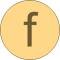# writeRealMatrix

Write Real matrix to a MATLAB MAT file# Information

This information is part of the Modelica Standard Library maintained by the Modelica Association.

#### Syntax

```success = Streams.writeRealMatrix(fileName, matrixName, matrix, append, format)
```

#### Description

Function writeRealMatrix(..) writes the given matrix to a new or an existing MATLAB MAT file (in format v4, v6, v7, and if HDF is supported in the Modelica tool, also v7.3). If `append = false` (= default), the file is newly created (or an existing file is deleted and re-created). If `append = true`, the matrix is included in an existing file or if the file does not yet exists this flag is ignored. If the file exists and `append = true`, argument format is ignored.

Parameter format defines the format in which the values are stored on file. The following formats are supported:

 format = Type of format "4" MATLAB MAT version v4 "6" MATLAB MAT version v6 "7" MATLAB MAT version v7 "7.3" MATLAB MAT version v7.3 (requires HDF support in the Modelica tool)

The function returns `success = true` if the matrix was successfully written to file. Otherwise, an error message is printed and the function returns with `success = false`.